Back to my homepage

# A paper/pencil game

1. Game description
2. Analysis
3. Combinatorial Game Theory
4. The game of nim
5. Grundy-Sprague theorem
6. Solving the 1*n game
7. Solving the full game
8. Variants
9. References

[prolog code]

### 1. Game description

Two persons play on some rectangular checkered board. Players alternate in putting a piece in a free square (just like in Go). If played on paper, they can just mark a square in every step. It is not allowed to choose a square that has any of its neighbors (out of the 8 or less) already taken. If a player can't put a piece anywhere, he loses.
The game is described at Paper&Pencil games, under the name "Obstruction Game". There it can also be played against the computer. Mathpuzzle featured it as "Obliteration Game".

 O X O X

Since the gameplay is completely deterministic, one of the players must have a winning strategy for each board size. For example, the first player wins on a 3*3 board, but loses on 2*4. For smaller boards it's trivial to figure out who wins, but on a large board it seems difficult to guess, and it's not clear how to find the optimal move in every position. As a puzzle, you can find who wins on 4*4, 4*5, 4*6, 5*6 and 6*6.

When I came up with the idea of this game, I didn't know of anything similar. Doing some research, I found several existing games that are closely related. The 1*n version is known as Dawson's chess, it can be played online here, and a game called Regio already exists, which is almost the same, the only difference being that in Regio only the 4 direct neighbors are considered, instead of 8.

### 2. Analysis

Veres Péter pointed out that on board sizes where both the height and width are odd numbers, the first player has a simple winning strategy: put first in the middle, then in each turn just "mirror" the second player's move (put in the square that is opposite to the other player's move with respect to the center of the board). With this strategy the first player will always have a place to put, while the second player will run out of free squares.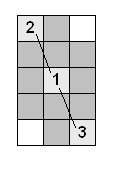In other cases it seems that there is no such "short-cut". On (even * even), in some cases the first player wins (2*2), in others the second (4*4).
The trick works on 1*n boards too. If the board size is odd, the first player can put in the middle first, and win, but for even board sizes there is no simple rule. For example, 4 is loser, but 6 is winner.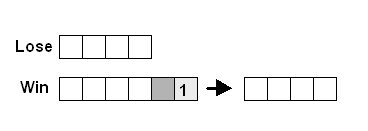Let's try to attack the problem with brute force. Each move can be thought of as cutting out a 3*3 piece from the board, with the current move in the center (this piece can be smaller, if it overlaps with the margin or already cut-out parts). We can construct a graph with every possible board configuration. We don't consider those that can be obtained through rotation/mirroring. Each board configuration is represented by a node, and edges show the possible moves of a player. Every board configuration (every node) can be labeled as 'Winner' or 'Loser', from the point of view of the player who's moving first in that situation.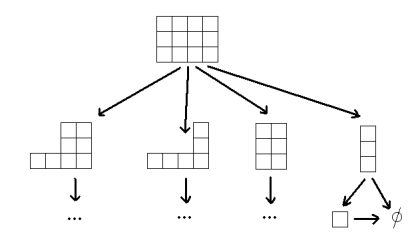Every path eventually leads to the empty position. This can be considered 'Loser' and from there we can trace back every other game configuration.
The rule for labeling a node is the following:

• a node is 'Loser', if all its successors are 'Winner' (no matter what move the player makes, the other player has a winning strategy).
• a node is 'Winner', if at least one of its successors is a 'Loser' (player can take that move, and force the opponent to lose)
The winning strategy is obvious: always make a move that leads to an L situation ('Loser' for the other player).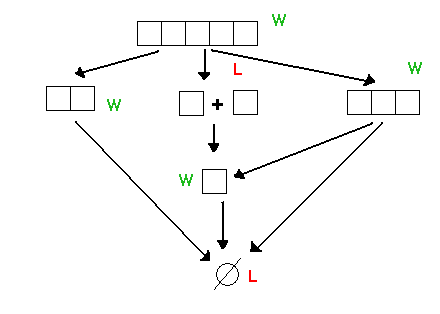With this method we can computationally solve the game (this is a special case of the well-known minimax-algorithm). But the solution doesn't feel quite right. As the game approaches its end, often the board will break-up into small disjoint 'islands'. With this method we have to remember every piece that makes up a full configuration. What if we knew the individual outcome of all these small boards. Could we tell anything about their total?

Let's see some special cases. In the following 1 will denote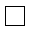and 3 will denote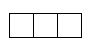We can observe:

• 1 + 1 + 1 is equivalent to 1, so it's a Win.
• 1 + 1 is equivalent to the empty board (a lost game).
• if A is Lose, 1 + A is Win.
• A + A is always Lose, since whatever move the first player makes, the second player can imitate it on the other board.
• two Lose games together are a Lose (if the first player moves on one board, the other player will move on the same board, forcing the first player to lose both boards one after the other).
• Win and Lose always make a Win.

What about two Win games? As it turns out, this information is not enough to tell the outcome of their sum. For example 1 and 3 alone are both Wins. But while 1 + 1 is Lose, 1 + 3 is Win. The key idea in 1 + 3 is that the first player can play on 3 as to lose it, reducing the configuration to 1 + 1 first, where he wins. Intuitively we feel that while 1 and 3 are both Wins, there is more to 3 than 'Win', on 3 the player is not forced to win, he can lose it if he wants, thus saving a valuable step elsewhere. We can see that this W/L classification doesn't capture the full complexity of the game situations, so we need some better tools.

Without taking any advantage of the fact that the board breaks up in smaller boards that can be solved separately, the running time of an algorithm is exponential with respect to the board size. Luckily we can do better than this using combinatorial game theory.

### 3. Combinatorial Game Theory

Combinatorial Game Theory(CGT) is the theory of two-player, perfect knowledge games. These games are based on the concept of a position, which is changed through the alternating moves of the two players, until some ending condition is satisfied. An ending position usually has an associated score, indicating which of the two players won, if any. In these games, both players have full information about the current position, as well as about the possible moves at any time in the future. The latter condition means that CGT does not study games that involve an element of chance. Typical games that can be studied using CGT are Chess, Go, Othello, etc.

An especially interesting class of combinatorial games is that of the finite impartial games. In these games it is guaranteed that the game ends after a finite number of steps. Impartial means that the moves of the two players are identical, a valid move by a player could be made by the other player, if it were his turn. The set of allowed moves depends only on the position, not on which player is currently moving. In this sense, Chess or Go are not impartial, because the players are constrained by the color of their pieces. As we can see, an impartial game is fully characterized by the set of positions, and the moves that make the transition from one position to the other. The gameplay can be represented by a finite, directed acyclic graph (DAG).

Impartial games end when there is an impossibility to move, usually the player who can not move loses. This is called normal play. In a different approach, the player who makes the last move loses. This is called misère play and it's much harder to analyze. We will consider normal play only. Needless to say, our paper/pencil game satisfies all the above conditions, so it's a finite impartial game with normal play.

An even simpler such game is nim, where the players have to pick objects from a heap of a certain size. The fundamental result of CGT states that every position in every finite impartial game is essentially equivalent to a certain game of nim. Since nim is easily solvable, the problem of solving a finite impartial game is reduced to finding the function that maps a game position to a nim pile-size. This number that can be associated to every position is also called "nimber", nim-value or Grundy-value.

### 4. The game of nim

In the game of nim, we have a pile with a certain number of objects, say pebbles. Players alternate in taking away any number of pebbles they want. If a player has no pebbles left, he loses. This game is trivial, of course, because the first player can pick up all the pebbles, and thus win instantly. For any size of the pile, the first player is the winner, except if the pile was empty to begin with, in which case he can be considered to have lost, even before the game starts.

To make the game more exciting, we can play simultaneously with several piles of pebbles. In each turn a player decides on which pile to play, and takes away pebbles only from that pile. The player who doesn't have any pebbles left loses. The winning strategy is not so obvious anymore. Take for example a pile of 2 and 3. What should the first player do? It turns out that his best move is to take one pebble away from the 3-pile. Now that it's 2+2, the player who moves loses because the other player can 'mirror' all his moves on the other pile.

A sample gameplay (source: Wikipedia)

 Heap-sizes Moves A B C 3 4 5 I take 2 from A 1 4 5 You take 3 from C 1 4 2 I take 1 from B 1 3 2 You take 1 from B 1 2 2 I take entire A heap 0 2 2 You take 1 from B 0 1 2 I take 1 from C 0 1 1 You take 1 from B 0 0 1 I take entire C heap and win.

While this version with many piles seems significantly more complex than the one-pile nim game, they are in fact equivalent. Remember that the single-pile game was loser for a zero pile-size, winner otherwise. A many-pile game also has a unique number (nim-value) associated to it, which we can find adding the pile-sizes using binary digital sum (bitwise-XOR). If this number is zero, the current position is loser, if it is different than zero, then there exists a move that reduces the nim-value of the game to zero. The winning move is always one that reduces the XOR-value of pile-sizes to zero. The proofs by induction can be found here.

### 5. Sprague-Grundy theorem

We now know that a game position corresponds to a pile-size in a simple nim game. In order to find a winning move if there exists one, all we need to do is find out the nim-value of the current position and the successor positions. In order to do this efficiently, we use the following two properties:

a) Sprague-Grundy theorem. The nim-value of a position is the minimum-excluded value of the nim-values of the successor positions. If we know the nim-value of all the positions that can follow from position P, then the nim-value of P is the smallest natural number that is not among the nim-values of the successors. A proof of this property and a more formal definition can be found here.

b) Adding game positions (nim-sum). This is analogous to the nim game played on many piles simultaneously. According to the theorem, if P1 and P2 are two game positions, having nim-values x1 and x2, the game P = P1 + P2 has a nim value x = x1 ⊕ x2. P1 + P2 refers to the game obtained by playing the games P1 and P2 simultaneously (in each turn a player decides in which game to make one move). In a special case, two or more identical positions always result in a sum with nim-value 0, bacause x ⊕ x = 0, therefore a losing position, as we knew it already. (⊕ stands for bitwise XOR)

These theorems allow us to efficiently calculate the nim-value of every game position in our original game. This nim-value gives a finer characterization of a position, than the simple Win/Lose scheme we used in 2. Positions with 0 nim-value correspond to the Lose positions, and all the non-zero natural numbers are Win positions. Now we can add two or more Win positions too, by calculating the ⊕ sum of their nim-values.

This method allows us to write a reasonably efficient program that can evaluate a position, without needing prohibitively many calculations or memory space. Unfortunately, for a human player, on a large board this is still not a "solution", since calculating the nim-value of a board still requires to analyze every possible successor of a position. For a human player this is probably too much to keep in mind, except for the 1*n game. The only value of these theorems for this game is that we can reuse small board sizes that have been already solved, and in the case when the board splits up into smaller boards, we can deal with each piece separately.

The fact that finding the solution is still computationally expensive, means that the game can indeed be enjoyable for people, unless some simple solution is found for the general case, similarly to the middle-point strategy for the (odd*odd) boards. While I can't rule out this possibility, the structure and complexity of the game positions suggests that it is unlikely that there is such "short-cut". At the moment, even writing a program that efficiently plays on an n*m board seems far from being a trivial task.

### 6. Solving the 1*n game

Let's find an efficient algorithm for solving the original game for an 1*n board.
In the following n denotes the board of size n, such as 3 forn->{n1,n2,...} means that n1 and n2 can result from a move in n. For example 4->{1,2}. We call these elements successors of n.
n+m refers to a position that consists of disjoint boards of sizes n and m. For example 5->{3,2,1+1}.
g(x) is the nim-value of a board of size x. Remember that a nim-value of 0 means that the board is a Lose position, a non-zero value indicates a Win position.

#### Finding out if board x is Winner/Loser

We have to find g(x).
First we calculate all the possible successors of board x:

• player can put in a margin, which leads to x-2.
• putting on the second square leads to x-3.
• putting anywhere else will result in A+B where A+B = x-3.

After obtaining the successors {A,B,C,...} we calculate the nim-value of each successor. If a successor is of the type A+B, we use the result that g(A+B) = g(A) ⊕ g(B).
After we find all the nim-values of the successors, g(x) is the minimum excluded value (mex) of these.
mex(S) is the smallest natural number not in S. ( mex(S) = min(N-S) )
In the end if g(x) = 0, x is Lose, if g(x) > 0, x is Win.

Let's see some examples:

x=0
g(0) = 0 by definition (the empty board is Lose)

x=1
1->{0} (the only possible successor of 1 is 0)
g(0) = 0.
g(1) = mex{0} = 1. (Win)

x=2
2->{0}
g(0) = 0.
g(1) = mex{0} = 1. (Win)

x=3
3->{0,1} (on a board of 3 we can put either in the middle, or on a margin)
g(0) = 0.
g(1) = 1.
g(3) = mex{0,1} = 2. (Win)

x=4
4->{1,2}
g(1) = 1.
g(2) = 1.
g(4) = mex{1,1} = 0. (Lose)

x=5
5->{2,3,1+1}
g(2) = 1.
g(3) = 2.
g(1+1) = g(1) ⊕ g(1) = 0.
g(5) = mex{0,1,2} = 3. (Win)

......

Following this idea it is fairly easy to write a program that solves an 1*n board. I wrote a simple program in prolog. The full code can be downloaded as nim.prolog (it should run in any prolog top-level, for example in gprolog). A portion of the code (the function that calculates g(x)) is here:

% Nim-value for a board of size n
:-dynamic(nim_value/2).
nim_value(0,0):-!.
nim_value(1,1):-!.
nim_value(X,N):-
successor(X,S),
solve_all(S,NS),
mex(NS,N),
asserta(nim_value(X,N)), % saving the nim-value for later use
!.

The lines follow the steps in the algorithm described above. The key idea is that once we find out the nim-value for a board-size x, we store it in the memory (the asserta command). This way, every board-size will be computed only once, the first time when it is needed. With this trick, we improved the running time from exponential to linear. In the same time, the memory space usage increased, but only to linear (each x is stored in memory once).

Without this trick, the largest board size that could be solved by the program was 28. With assert solving a board size of 800 takes less than a second.

Using this program we can find if a board is Win/Lose:

| ?- solve(40,X).
X = 'Win'

If we want to display the nim-values sequence for board sizes 1..n, we can do it as follows:

| ?- grundy(20,X).
X = [[0,0],[1,1],[2,1],[3,2],[4,0],[5,3],[6,1],[7,1],[8,0],[9,3],[10,3],[11,2],[12,2],[13,4],[14,0],[15,5],[16,2],[17,2],[18,3],[19,3],[20,0]]

The sequence is 0,1,1,2,0,3,1,1,0,... I was curious if this sequence has any particular importance, and checked it in the Encyclopedia of integer sequences. The surprising response was:

A002187 Sprague-Grundy values for Dawson's Chess

It was in this way that I learned that the game already exists.

#### Finding a good move

Now that we have the winning positions, we can make the program play a game. The difference is that now we might already be in an A+B position where the board has been split up into smaller pieces. We have to consider all successors of this position, so we have to take each separate case when the player makes a move on one particular portion.

The code that makes the pick is the following:

% Pick one element after another from a list
pick([H|_],H).
pick([_|T],X):-pick(T,X).

pick() is non-deterministic, which means that if the choice doesn't lead to a good move, it can be back-tracked.
If there is one element among the successors with nim-value 0, we have to move in that direction, because that's a Lose for the opponent.

An example run (program gives next position after a good move):

| ?- next_move(,X).
X = [2,7]

| ?- next_move([1,2,3],X).
X = [1,2,0]

| ?- next_move(,X).
X = 'No good moves, this is a Lose position.'

### 7. Solving the full game

The full game is still far from being solved. Along similar reasoning as for the 1*n game, it should be possible to write a program that plays on reasonable board sizes (say, up to 8x8). As for human play, I am not aware of any tricks that would give a simple strategy in the general case.

For a program that plays the game, one would supposedly need some data structure to hold different shapes of various sizes. For each shape we can calculate the nim-value as before. At least for smaller sizes, a lot of the nim-values should be precomputed. For each shape we can find the successor values. If the board breaks up into smaller boards, we solve the issue in the same way as in the 1*n case.

To optimize calculations, shapes with rotational and mirror symmetry can be considered the same. If the board reaches an odd*odd size, the winning strategy should be used from there. However, this doesn't work if the board is an odd*odd piece, plus other pieces. In this case we still need to know the exact nim-value of each piece.

In order to make the algorithm efficient, further ideas would be needed. At this point, I don't see any significant improvements on this approach. Any suggestions are welcome.

### 8. Variants

I was trying to think of more or less natural generalizations of the game:

• the sides of the board touching each other. If you put a piece next to the margin, this eliminates the 'neighboring' squares on the other side too. This is equivalent with the board being a torus. Is the game-strategy different than before?
• in theory, the game could be played on other topologies as well, such as a Mobius strip
• instead of rectangular grid we could use a hexagonal or any other tiling
• the board can be 3D

While these generalizations seem to become more and more complex, I realized that there is a simple version that is basically equivalent to all of the above:

Two players playing on an arbitrary graph, where they color vertices in turns, and it is not allowed to pick a vertex that has any of it's neighboring vertices already colored. The neighborhood information is captured in the edges of the graph. This is a finite impartial game that can be studied similarly to the original one.

This is also a version of the famous map-coloring problem, which asks how many colors are needed to paint each region of a map in a way that neighboring regions have different colors. This can be played as a two player game, where the first player who is not able to color any region with a valid color loses. Our game is just the special case of the map coloring game, where the number of colors is 1. I found that this coloring game with a single color on arbitrary maps was published in 1971 in David Silverman's book 'Your Move'. It is called 'Monochrome' and our initial paper/pencil game is merely a special case of it.

Many puzzles can be reduced to graph-coloring problems. For example, the popular Sudoku game practically asks for the coloring of a special graph with 9 different colors(numbers), where some of the vertices have already been colored.

### 9. References

Wikipedia: Sprague-Grundy theory
Wikipedia: Nim
Wikipedia: Impartial game
Wikipedia: Nimber
Wikipedia: CGT
Mathworld: Nim-value
AMS article on Nim
The Sprague-Grundy theory of impartial games
Yuval Peres - Game Theory lecture notes (UC Berkeley)
Thomas S. Ferguson - Game Theory lecture notes (UCLA)
John Horton Conway: On Numbers and Games (Academic Press Inc, 1976)
Dawson's chess
Regio